# HSPT Math : Concepts

## Example Questions

### Example Question #61 : Negative Numbers

Fill in the blank with the proper sign.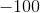__________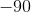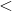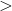Explanation:

In order to solve this problem, we will use a number line. Numbers to the left of zero on the line are less than numbers to the right.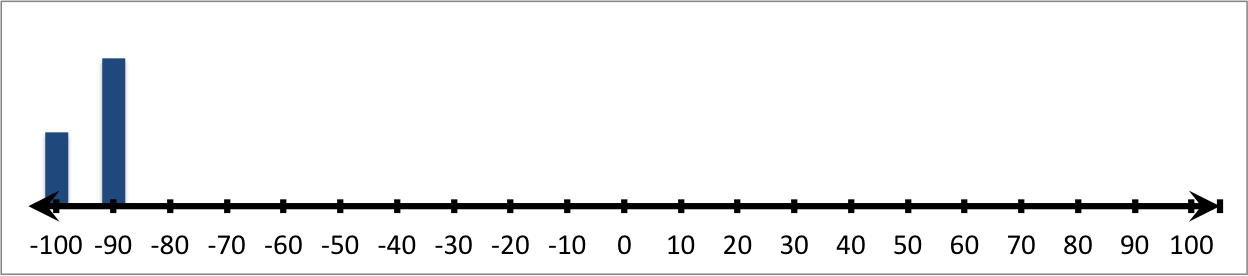Therefore: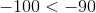### Example Question #21 : Understand An Inequality On A Number Line: Ccss.Math.Content.6.Ns.C.7a

Fill in the blank with the proper sign.__________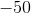Explanation:

In order to solve this problem, we will use a number line. Numbers to the left of zero on the line are less than numbers to the right.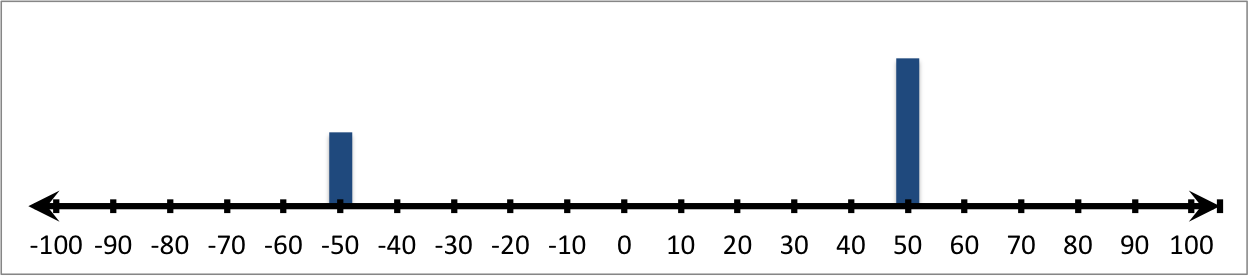Therefore: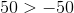### Example Question #61 : Concepts

Fill in the blank with the proper sign.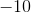__________Explanation:

In order to solve this problem, we will use a number line. Numbers to the left of zero on the line are less than numbers to the right.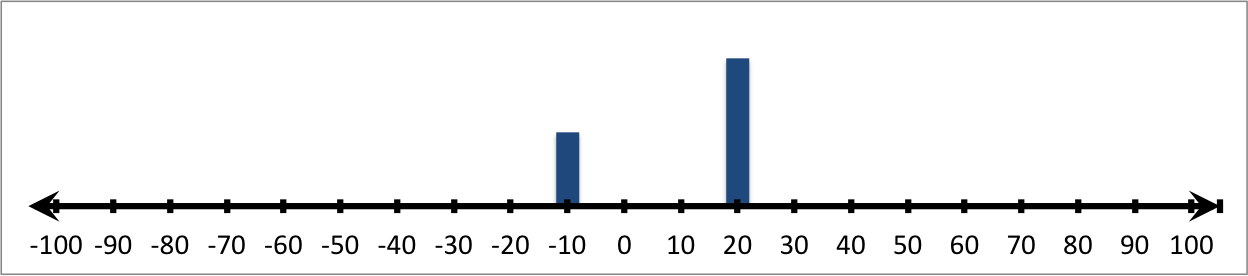Therefore: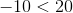### Example Question #21 : Understand An Inequality On A Number Line: Ccss.Math.Content.6.Ns.C.7a

Fill in the blank with the proper sign.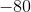__________Explanation:

In order to solve this problem, we will use a number line. Numbers to the left of zero on the line are less than numbers to the right.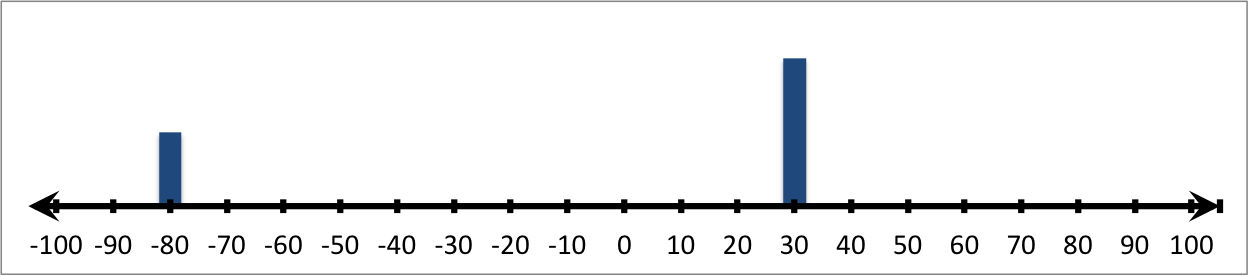Therefore: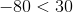### Example Question #474 : The Number System

Fill in the blank with the proper sign.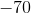__________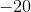Explanation:

In order to solve this problem, we will use a number line. Numbers to the left of zero on the line are less than numbers to the right.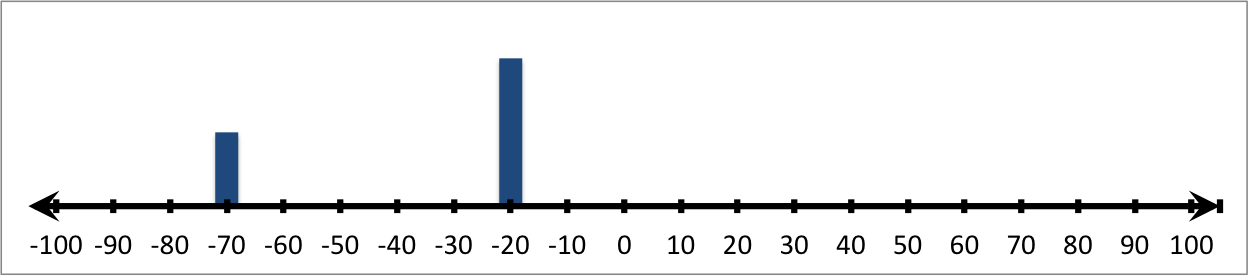Therefore: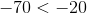### Example Question #61 : Concepts

Fill in the blank with the proper sign.__________Explanation:

In order to solve this problem, we will use a number line. Numbers to the left of zero on the line are less than numbers to the right.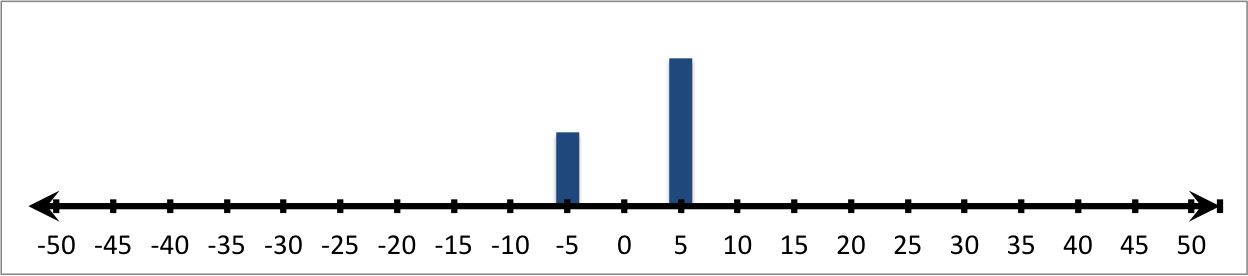Therefore: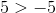### Example Question #67 : Concepts

Fill in the blank with the proper sign.__________Explanation:

In order to solve this problem, we will use a number line. Numbers to the left of zero on the line are less than numbers to the right.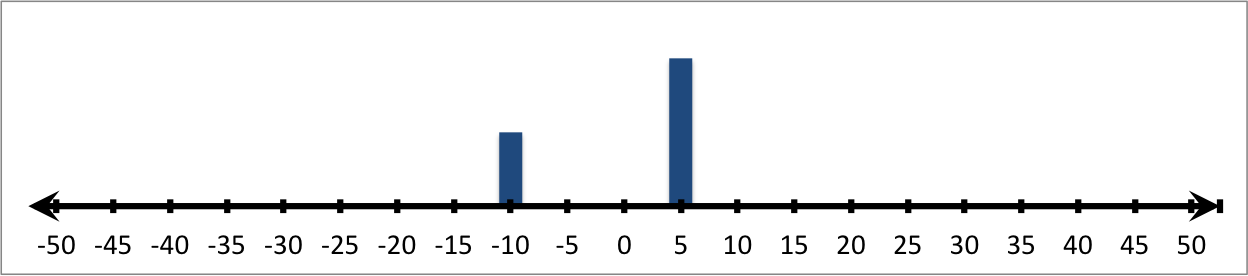Therefore: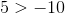### Example Question #562 : Grade 6

Fill in the blank with the proper sign.__________Explanation:

In order to solve this problem, we will use a number line. Numbers to the left of zero on the line are less than numbers to the right.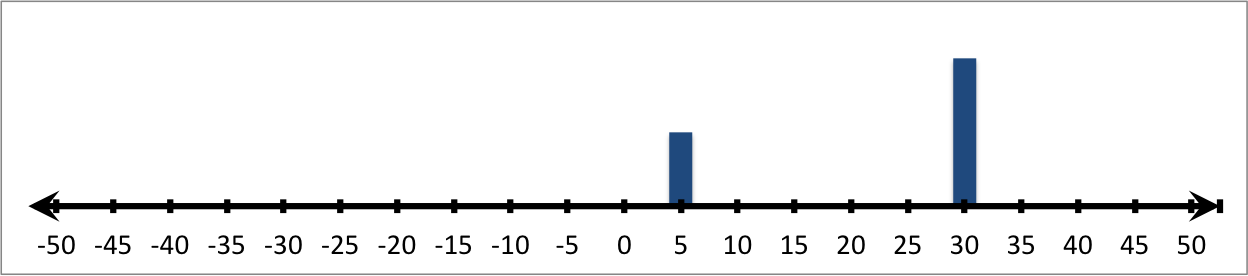Therefore: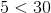### Example Question #62 : Concepts

Fill in the blank with the proper sign.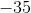__________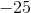Explanation:

In order to solve this problem, we will use a number line. Numbers to the left of zero on the line are less than numbers to the right.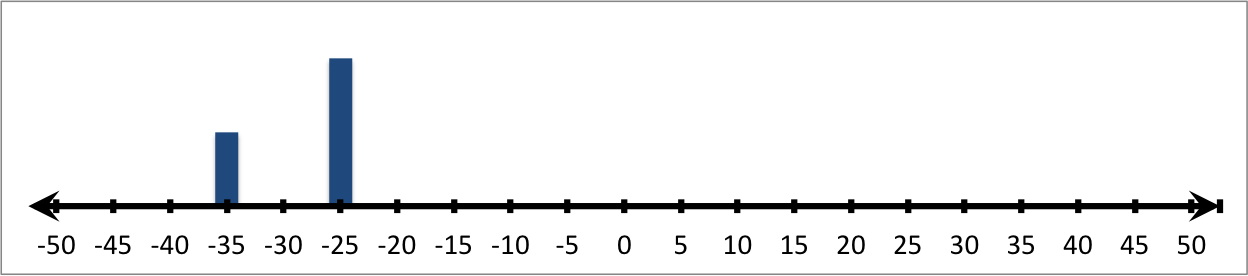Therefore: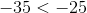### Example Question #62 : Concepts

Fill in the blank with the proper sign.__________Explanation:

In order to solve this problem, we will use a number line. Numbers to the left of zero on the line are less than numbers to the right.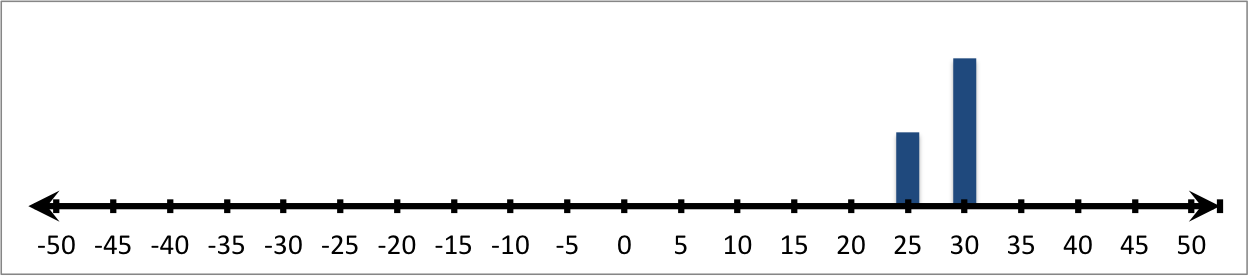Therefore: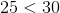### All HSPT Math Resources